# 海上钻井中运用泥浆温度测算地温梯度方法研究Estimation of True Formation Temperature Based on Mud Temperature Data in Offshore Drilling

DOI: 10.12677/JOGT.2020.421005, PDF, HTML, XML, 下载: 109  浏览: 810

Abstract: Ultra-high temperature well appears in Baiyun sag. It is predicted that the temperature range of the formation in the sweet spot section is 200˚C~250˚C, which has reached or even exceeded the temperature tolerance limit of most logging tools, bringing great challenges to the exploration operation. Under the condition that the tool temperature resistance cannot be improved in a short period of time, accurate formation temperature measurement is the basis of high-temperature well operation scheme design and operation tracking decision-making. The traditional calculation method introduces many parameters and some parameters are difficult to obtain. It has poor ap-plicability for the new target and the block with a lack of test temperature data, so it cannot solve the operation problem quickly. In this paper, two new methods of using mud temperature to cal-culate formation temperature are put forward by simplifying the model and summarizing the law of multi-well data. The method has the characteristics of refining calculation parameters and strong applicability. The new method has been used in BY2-F well and good results have been obtained.

1. 引言

2. 传统计算方法(Hornor图版法)

${T}_{f}={T}_{o}+\left(\frac{H}{4\pi \phi }\right)×\mathrm{ln}\left[1+\left(\frac{{t}_{c}}{{t}_{s}}\right)\right]$ (1)

3. 两种单井预测地温的新方法

3.1. 井底单点静止泥浆温度回归法(方法1)

3.1.1. 方法思路及计算步骤

${T}_{oti}={e}^{{\beta }_{1}+{\beta }_{2}{t}_{si}}\left(i=1,2,3,\cdots ,n\right)$ (2)

${T}_{oti}={T}_{oi}+\left({h}_{b}-{h}_{oi}\right){G}_{c}\left(i=1,2,3,\cdots ,n\right)$ (3)

${G}_{c}={T}_{b}-{T}_{sb}/\left({h}_{b}-{h}_{sb}\right)$ (4)

${T}_{b}={T}_{otm}\left(当{t}_{s}趋向于无穷大时\right)$ (5)

① 电缆测井时测得i组静止泥浆温度数据，( ${T}_{o1}$${t}_{s1}$ ) ( ${T}_{o2}$${t}_{s2}$ ) $\cdots$ ( ${T}_{oi}$${t}_{si}$ )。

② 根据(3)式，给 ${G}_{c}$ 取一个初始值(可根据地质设计中的区域预测值)，将步骤①中测得的数据换算成井底温度和静止时间的组合( ${T}_{ot1}$${t}_{s1}$ ) ( ${T}_{ot2}$${t}_{s2}$ ) $\cdots$ ( ${T}_{oti}$)，用这几组数据根据(2)式方程进行回归，求得 ${\beta }_{1}$${\beta }_{2}$

③ 在(2)式中令 ${t}_{s}$ 趋向于无穷大，求得 ${T}_{otm}$，即 ${T}_{b}$

④ 将 ${T}_{b}$ 代入(4)式求得 ${{G}^{\prime }}_{c}$，当 $|{{G}^{\prime }}_{c}-{G}_{c}|\ge 0.01$ 时，令 ${G}_{c}={{G}^{\prime }}_{c}$，返回第②步，进行迭代计算。

⑤ 当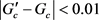时，迭代结束，此时最后一次计算中所得 ${T}_{b}$${G}_{c}$ 即最终计算结果。

3.1.2. 应用经验及影响因素分析

1) 至少3个可靠数据点回归出来的方程才具有可参考性。可知该方程本质是S型曲线，在实际应用中，主要关注当 ${t}_{s}$ 泥浆静止时间趋向于无穷大时的温度极限值，即曲线的右半部分形态，当 ${t}_{s}$ 趋向于0时，方程并没有对应的实际意义；因此，需要3个以上的数据点才可以较好的刻画出曲线右半部分的形态。在实际应用过程中，3个数据点可以提供较为可靠的预测结果，4个及以上的数据点则计算结果精度较高。

2) 油基泥浆运用该方法进行计算时，所得计算结果可能会明显小于地层真实温度。油基泥浆比热容相对水基泥浆小，钻进时油基泥浆的温度会更高，因此停泵时的起始泥浆温度更高。导致拟合出来的曲线方程右半部分较水基斜率更缓，从而得到相对较低的温度值。通过统计2次油基泥浆井的计算结果，最终计算温度较实际地层温度低了3.2%和4.5%。

3.2. 不同井眼的循环泥浆温度梯度回归法(方法2)

3.2.1. 循环泥浆温度的2个特点

1) 每个井眼的循环泥浆温度与井深呈线性关系。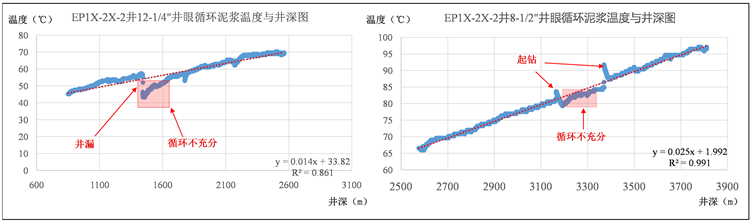Figure 1. Temperature gradient diagram of circulating mud under different working conditions

2) 不同井眼拟合出来的直线斜率(即循环泥浆温度梯度)不同；且呈现井眼越小，梯度越大的规律。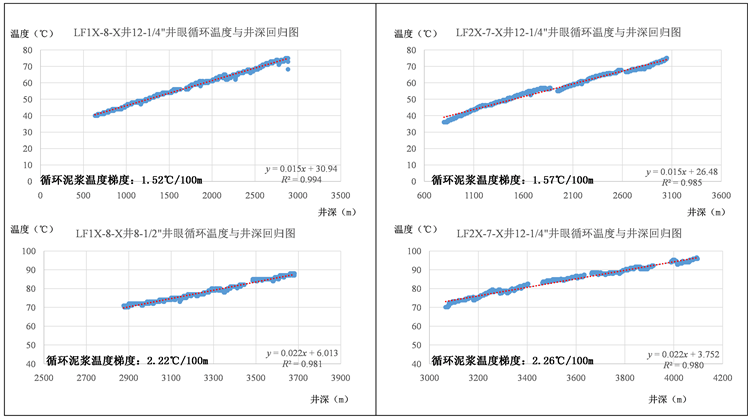Figure 2. Comparison of temperature gradients of circulating mud in different holes at the same well

3.2.2. 方法思路及计算步骤

${G}_{m}={\beta }_{3}{e}^{{\beta }_{4}{S}_{h}}$ (6)

3.2.3. 应用经验及影响因素分析

1) 回归出来的曲线方程，R2应至少大于0.95，如R2 < 0.95，则应根据工程日报剔除去无效点之后，再进行拟合。当数据样本较好且筛选得当，多数情况下得到的R2 > 0.98，此时计算结果较准确。

2) 当水深超过300 m，泥浆池升温梯度与循环泥浆温度梯度之比小于0.4时，可直接应用该方法进行计算；否则，则应考虑对排量、ROP变量进行校正。该模型选取井眼截面积作为影响降温效果的决定性因素，隐含了一个前提条件即泥浆循环中降温系统能力远大于升温系统，只有满足上述条件，排量、ROP和井深的影响因素才可以忽略；如降温能力没有远大于升温能力，随着井深变深，升温系统的能力逐步增大，那么方法2模型中动态平衡的基础就没有了。因此水深越深，该方法的应用效果越好；换言之，方法2在深水区钻井有良好的应用效果。

4. 测压取样温度数据的局限性

1) 理论上，测压取样作业是要求从浅层至深层依次进行；而实际作业过程中，由于各深度点物性和井眼规则程度不同，测压取样所需的时间和成功率是不同的。在争分夺秒的测压取样作业中，跳过作业相对复杂的深度点，优先完成流度大、井眼规则的深度点是降低作业风险、提高作业时效的有效手段。这样的作业方式，势必无法保证测取温度记录时由浅至深的规律性，仪器上提下放、降温升温的过程中测得的温度准确性会大大降低。

2) 仪器上的温度计由于是升温调校，由低温环境变换到高温环境得到的数据准确度高；而从高温到低温时，温度计则非常不敏感。

3) 电子电路的温漂和线圈系的温度形变也会在不规律的升降温中产生更多的影响 。

5. BY2-F井实例应用

BY2-F井是白云凹陷钻探的一口深水井，该井水深814.8 m，泥面深度844.1 m，海底温度5.9℃。实钻过程中尝试运用方法1和方法2测算地温梯度，为后续电缆测井、DST测试作业准备提供决策数据。

BY2-F井为一口五开井，三开、四开、五开井眼分别为12-1/4井眼、8-1/2井眼和6井眼。

5.1. 方法1应用Table 1. Temperature data sheet of static mud in each hole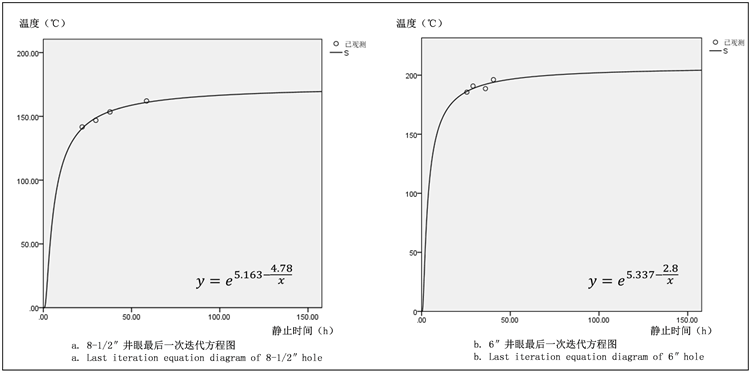Figure 3. Last iteration equation diagram of method 1Table 2. Summary of calculated geothermal gradient data

5.2. 方法2应用Table 3. Temperature gradient data of circulating mud in each hole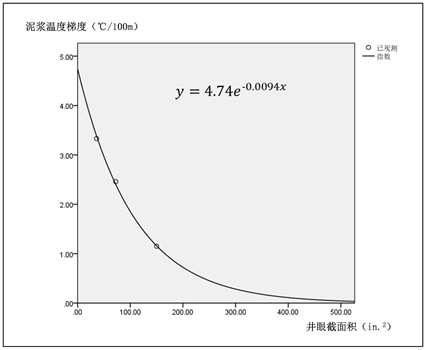Figure 4. Regression equation chart of method 2

5.3. DST作业实测温度

6. 结论

1) 从快速指导作业的角度出发，设计了2种利用泥浆温度测算地层温度的新方法，可以在钻井期间运用泥浆温度快速推算地层真实温度，为作业即时决策提供依据；在BY2-F井中进行运用，取得了良好的效果。

2) 从公式原理可以推论，方法1采用电缆测井期间测量得到的静止泥浆温度，影响温度变化的主要因素为静止起始温度和地层传热，受影响因素较少，因此可应用场景较多，无论在深水井和浅水井都有较高的准确率。

3) 方法2采用钻进期间的循环泥浆温度，该温度受钻井参数、海水深度、地层传热等较多复杂因素影响，简化模型的成立更依赖足够的海水降温能力，因此更适合在深水钻井中应用。如将方法2模型应用于浅水井的计算，需在原模型基础上，考虑用排量和ROP数据对原始温度数据进行校正。

  王界益, 高秋涛. 超高温高压井测井技术及应用[J]. 测井技术, 2008, 32(6): 556-561.  庞雄, 施和生, 朱明, 颜承志, 刘军, 朱俊章, 柳保军. 再论白云深水区油气勘探前景[J]. 中国海上油气, 2014, 26(3): 23-29.  米立军, 袁玉松, 张功成, 胡圣标, 何丽娟, 杨树春. 南海北部深水区地热特征及其成因[J]. 石油学报, 2009, 30(1): 27-32.  Fertl, W.H., Chilingarian, G.V. and Yen, T.F. (1986) Determination of True Static Formation Temperature from Well Logs. Energy Sources, Part A: Recovery, Utilization, and Environmental Effects, 8, 277-288. https://doi.org/10.1080/00908318608946056  Zare-Reisabadi, M., Kamali, M.R., Mohammadnia, M. and, Shabani, F. (2015) Estimation of True Formation Temperature from Well Logs for Basin Modeling in Persian Gulf. Journal of Petroleum Science and Engineering, 125, 13-22. https://doi.org/10.1016/j.petrol.2014.11.009  I.M. Kutasov, 李兰忠. 用经验公式估算地温梯度[J]. 国外油气勘探, 1995, 7(6): 782-783.  Cheng, W.-L., Huang, Y.-H., Liu, N. and Ma, R. (2012) Estimation of Geological Formation Thermal Conductivity by Using Stochastic Approximation Method Based on Well-Log Temperature Data. Energy, 38, 21-30. https://doi.org/10.1016/j.energy.2011.12.047  王力峰, 陆敬安, 沙志彬, 梁金强. 利用非平衡态温度测井数据估算地温梯度[J]. 地质科学, 2014, 49(3): 864-873.  何世明, 张明江, 唐长书, 丁云杰, 卞先孟, 张冠军. 用电测井眼温度估算原始地温的方法研究[J]. 天然气工业, 2005(2): 64-66+207-208.  李卫卫, 饶松, 唐晓音, 姜光政, 胡圣标, 孔彦龙, 庞菊梅, 王建存. 河北雄县地热田钻井地温测量及地温场特征[J]. 地质科学, 2014, 49(3): 850-863.  唐晓音, 黄少鹏, 杨树春, 姜光政, 胡圣标. 南海珠江口盆地钻井BHT温度校正及现今地温场特征[J]. 地球物理学报, 2016, 59(8): 2911-2921.  任敏, 马火林, 王文娟, 张祎然, 唐杰, 王建伟. 井筒中温度场测井响应特征模拟[J]. 工程地球物理学报, 2014, 11(1): 71-76.  杨谋, 孟英峰, 李皋, 邓建民, 张林, 唐思洪. 钻井液径向温度梯度与轴向导热对井筒温度分布影响[J]. 物理学报, 2013, 62(7): 537-546.  何昊男, 韩明, 杨继宝, 丁志康. 空气中水体温度下降随时间的变化模型[J]. 电子世界, 2017(9): 117.  杨惠中. 简易井温曲线的近似稳态校正方法探讨[J]. 能源技术与管理, 2007(3): 48-50.  李国玉, 马明学, 彭智. 阵列感应测井仪温度校正方法研究[J]. 石油仪器, 2012, 26(4): 87-89.